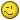Dividing a Total with criteria...

G

Guest

I am trying to write a formula that will figure out how many sections will go
into a number. The sections have to be between 10 and 20 feet each. For
example: If I have a 40 foot section, 2 20 foot sections will go evenly. If
I have a 50 foot section, 2 20 foot sections and 1 10 foot section.
Moreover, for a 25 foot section, it has to be 2 12.5 foot sections or 1 10
foot section and one 15 foot section, not 1 20 foot section and 1 5 foot
section, because all sections have to be between 10 and 20 feet.
So, each section has to be between 10 and 20 feet and I am trying to get as
few number of sections as possible.

Anyone have any idea how to accomplish something crazy like this with a
formula or even in multiple steps?

H

HighTide

Try this
If your total length is in A1
B1> =IF(MOD(A1,20)<10,"2x"&(20+MOD(A1,20))/2,"1x"&MOD(A1,20))&"'"
C1> =IF(MOD(A1,20)<10,QUOTIENT(A1-20,20),QUOTIENT(A1,20))&"x20'"

If you have 20' sections, you'll have only one or two cuts, if two
they will be equal length

B

BobT

My previous gives you 2 10's if your total is an exact multipe of 20,
sorry. This fixes that.

B1>=IF(MOD(A1,20)=0,"",IF(MOD(A1,20)<10,"2x"&(20+MOD(A1,20))/2,"1x"&MOD(A1,20))&"'")
C1>=QUOTIENT(A1,20)-IF(OR(MOD(A1,20)=0,MOD(A1,20)>=10),0,1)&"x20'"

G

Guest

Thank you very much!

BobT said:
My previous gives you 2 10's if your total is an exact multipe of 20,
sorry. This fixes that.

B1>=IF(MOD(A1,20)=0,"",IF(MOD(A1,20)<10,"2x"&(20+MOD(A1,20))/2,"1x"&MOD(A1,20))&"'")
C1>=QUOTIENT(A1,20)-IF(OR(MOD(A1,20)=0,MOD(A1,20)>=10),0,1)&"x20'"

B

BobT

Any time, as long as you're wiling to put up with my
revisions.
Note that the latest I gave you still have bugs if your
total is <10'. I won't bother revising as that is outside
your original parametersG

Guest

This worked out perfect. I'm glad my crazy question was understandable.